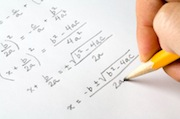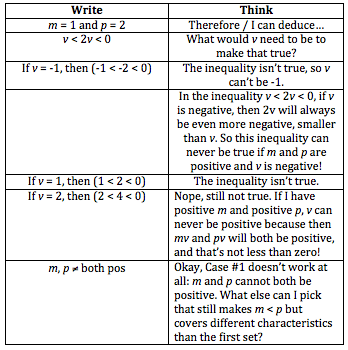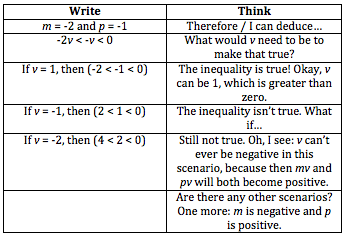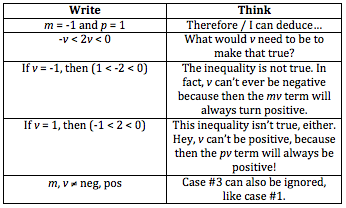# The 4 Math Strategies Everyone Must Master - Part I

by , Dec 23, 2013We need to know a lot of different facts, rules, formulas, and techniques for the quant portion of the test, but there are 4 math strategies that can be used over and over again, across any type of mathalgebra, geometry, word problems, and so on.

Do you know what they are?

Try this GMATPrep problem and then well talk about the first of the four strategies.

* If mv < pv < 0, is v > 0?

(1) m < p

(2) m < 0

All set?

How did you do the problem? Most quant questions have more than one possible approach and this one is no exceptionbut I want to use this problem to talk about a particular technique called Testing Cases.

This question is called a theory question: there are just variables, no real numbers, and the answer depends on some characteristic of a category of numbers, not a specific number or set of numbers. When we have these kinds of questions, we can use theory to solvebut that can get very confusing very quickly. Instead, try testing real numbers to prove the theory to yourself.

(Note: I chose a particularly tough question for this exercise; testing cases can also be useful and fast on easier questions!)

This problem gives one inequality:

"mv < pv < 0"

The test writers are hoping that youll say, Oh, lets just divide by v to get rid of it, so the equation is really m < p < 0. But thats a trap! Why?

When you divide an inequality by a negative, you have to flip the signs. But you dont know whether v is positive or negative, so you dont know whether to flip the signs! Never divide an inequality by a variable if you dont know the sign of the variable.

The question itself contains a clue (two, actually!) pointing to this trap. The given inequality asks about < 0 and the question also asks whether v > 0? Less than zero and greater than zero are code for Im testing you on positives and negatives.

So, we cant actually simplify that annoying inequality. Things are going to get a little messy, but can you figure anything out to start? Glance at that starting inequality for a second: what cant the value be? None of the variables can be 0, or the inequality mv<pv< 0would be false.

Do you want to start with statement 1 or statement 2? Since Im writing this, I get to choose (I want to start with statement 2), but there isnt one right answer to that question. Start wherever you want!

(2) m < 0

Hmm, m is negative. Call that -1. Then v could be -1 oh, wait, no v cant be negative. Then mv would be positive but mv is supposed to be less than 0. And I already said v cant be 0. If m is negative, then the only way to make mv negative is to make v positive. Cool!

Ive just proven the theory to myself by using a concrete number: I want mv to be negative. A negative times a positive equals a negative. If m is negative, then v has to be positive.

Statement 2 is sufficient. Cross off answers ACE.

(1) m < p

(This one gets messy: I suggest you write down the steps yourself as we go.)

Case #1: positive / positive.Case #2: negative / negative.Case #3: negative / positive.The only scenario that worked was case #2, and the only values for v that worked were positive. Therefore, v is greater than zero; the statement is sufficient.

As I mentioned earlier, the Testing Cases strategy can be used to make the work faster on lots of easier problems as well. In the case of these annoying theory questions, Testing Cases can really save us by making it (somewhat) easier to figure out whats going on with the theory.

For Strategy #2, Im going to send you to an article I wrote a long time ago: How to Turn Algebra into Arithmetic. (We officially call this strategy Choose Smart Numbers.)

This classic Problem Solving (PS) strategy has been around since the beginning of time (well, Standardized Test Time, anyway). It will work only on Problem Solving questions, not Data Sufficiency, but it is based on a similar principle: pick some real numbers to turn annoying algebra / variables / abstract math into a much more concrete problem.

One main difference exists between the two strategies discussed so far. When choosing smart numbers, you will only have to test one set of numbers in the problem. When testing cases on theory problems, you will almost certainly have to test multiple sets of numbers (as we did in the problem above).

Okay, begin! Ill start you out with a couple of easier ones from OG13. Note: always learn new techniques on easier problems first! You might not even choose to use the special technique on this problem during the real test, but thats the point: you'll learn how to use the technique more easily when you already understand how the textbook math works.

Test Cases: PS #32, PS #44, DS #1, DS #7

Choose Smart Numbers: PS #34, PS #38

Start thinking about how to recognize when you can use these strategies (how do you know?), and then practice them in different scenarios and across different content categories. Eventually, you will master these 2 essential techniques!

Finally, dont forget to join me next time, when well discuss the other 2 essential quant techniques that everyone must master.

* GMATPrep questions courtesy of the Graduate Management Admissions Council. Usage of this question does not imply endorsement by GMAC.